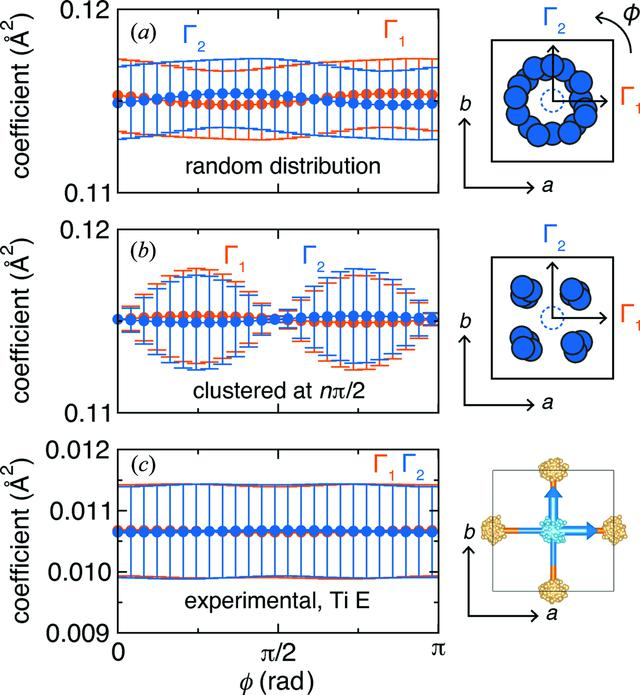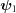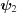disable zoom     view article Figure 6 Euler angle analysis of a multidimensional tight-binding mode set that transforms as a multidimensional irreducible representation. (a) A random distribution of atom positions from the crystallographic location (dashed circle in cartoon) produces an equivalent variance of the mixing coefficients (denoted with vertical bars) between the two basis vectors (and) when the basis vectors are rotated about the Euler angle, ϕ, parallel to the C4 axis of the crystal structure. (b) A clustering of positions at regular intervals, such as π/2, will produce the same mixing coefficients as in part (a) for each tight-binding mode when averaged over all k and over all ensembles. However, a clustering of positions will yield a significant variance in the coefficients, denoted by the vertical bars. (c) The coefficients provided from the experimentally derived BaTiO3 ensembles do not display significant differences when the basis vectors are rotated about the Euler angle.JOURNAL OFAPPLIEDCRYSTALLOGRAPHY
ISSN: 1600-5767
Volume 48| Part 5| October 2015| Pages 1560-1572